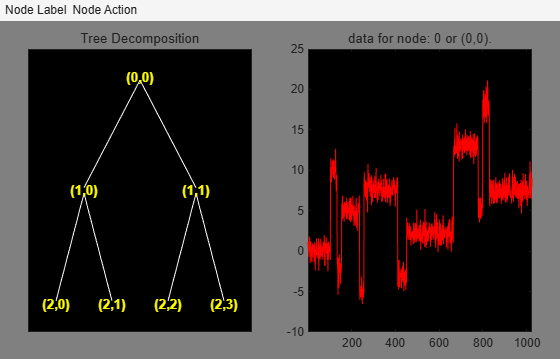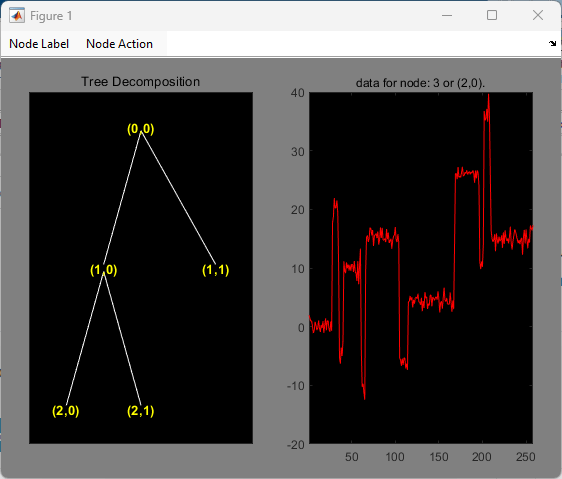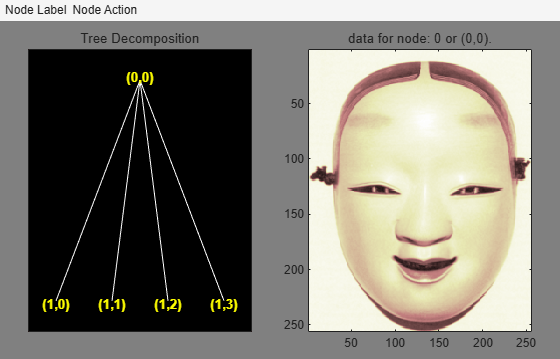plot

Description

plot is a graphical tree-management utility.

example

plot(t) plots the tree t. The figure that contains the tree is a GUI tool.

• You can change the Node Label to Depth_Position (default) or Index.

• You can change the Node Action to Visualize (default) or Split-Merge.

You can click the nodes to execute the current Node Action.

fig = plot(t) returns the handle to the figure.

newt = plot(trobj,'read',fig) returns the tree plotted in fig. You can use this syntax to return the tree after performing some split or merge actions.

Examples

collapse all

This example shows how to plot wavelet packet trees.

Obtain the wptree object that corresponds to a level 2 wavelet packet decomposition of the signal using the db2 wavelet.

x = noisbloc;
t = wpdec(x,2,'db2');

Plot the tree.

plot(t)Change Node Label from Depth_Position to Index. Click node (3) to obtain the following figure.Set the Node Label back to Depth_Position. Change Node Action to Split-Merge. Click on the (1,1) node to get the following figure. The figure shows the discrete wavelet transform down to level 2.Load an image. Obtain the wptree object that corresponds to a level 1 wavelet packet decomposition of the image using the sym4 wavelet.

t = wpdec2(X,1,'sym4');

Plot the tree.

plot(t)Change Node Label from Depth_Position to Index. Click the node (1). You get the following figure.Input Arguments

collapse all

Tree, specified as an ntree, dtree, or wptree object.

Object, specified as an NTREE-parented object. trobj is an object constructor name returning an NTREE-parented object.

Output Arguments

collapse all

Figure containing plot, returned as a Figure object.